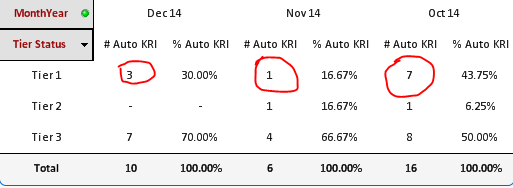# QlikView App Dev

Discussion Board for collaboration related to QlikView App Development.

Announcements
Join us at the Cloud Data and Analytics Tour! REGISTER TODAY
cancel
Showing results for
Search instead for
Did you mean:Creator II

## Count if Expression in Qlikview

Hi Dear Experts,

Got confused to write formula

Syntax :

if RAG_RATING_CD ='R'  only for current month count(Mar'16), not included previous two months(FEB'16 & JAN'16)

if RAG_RATING_CD ='R'  only if Two Months month count(FEB'16) equal, not included JAN'16 Count

if RAG_RATING_CD ='R'  if all three months values should be Equal, then we have to calculate the count

im using below expression which is not usful for me

please help me to get exact expression

=Count({<Month=, MonthYear={"\$(=Date(\$(vMonth),'MMM YY'))"},

MonthYear-={"\$(=Date(\$(vMonth)-10,'MMM YY'))"},

MonthYear-={"\$(=Date(\$(vMonth)-50,'MMM YY'))"}>} if ((RAG_RATING_CD) = 'R', 'Tier1'))

Thanks In Advance

Niranjan

2 RepliesPartner

you want to calculate the count for the union of all of the above conditions?

Your syntax is read as follows:

count the Tier1 where MonthYear is current Month and MonthYear is not 10 months back and MonthYear is not 50 months back

in the same set of data this is not logical

then you need to have this condition in multiple sets of data and use the union

=Count(

{

<Month=, MonthYear={"\$(=Date(\$(vMonth),'MMM YY'))"}>

+

<Month=,MonthYear-={"\$(=Date(\$(vMonth)-10,'MMM YY'))"}>

+

<Month=,MonthYear-={"\$(=Date(\$(vMonth)-50,'MMM YY'))"}>} if ((RAG_RATING_CD) = 'R', 'Tier1'))

hope this helps

I can walk on water when it freezesCreator II
Author

Hi Ali,

its Giving total count of Tier1 =3

but the same metric available in previous months as well, This scenario count should be less =2

i want to calculate only current months  Tier1 count, which is not included in previous month Tier1 (actual count is 2)Best Regards,

Niranjan